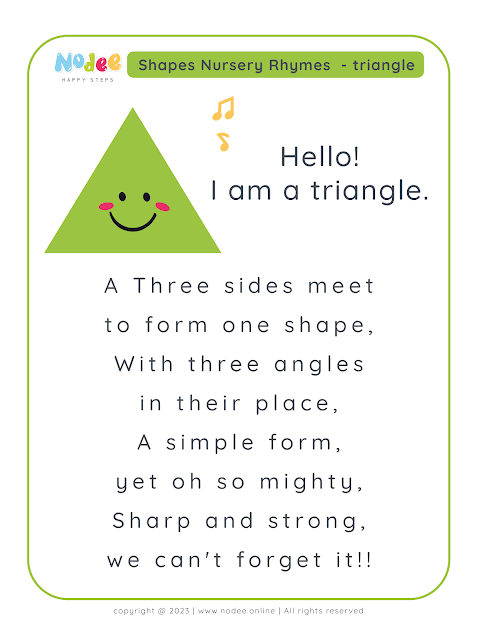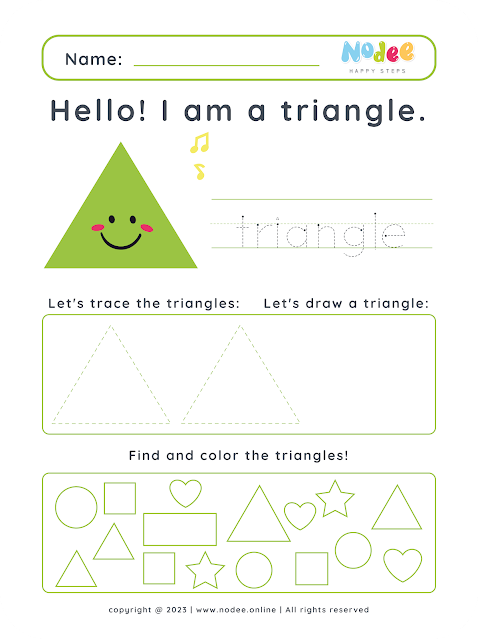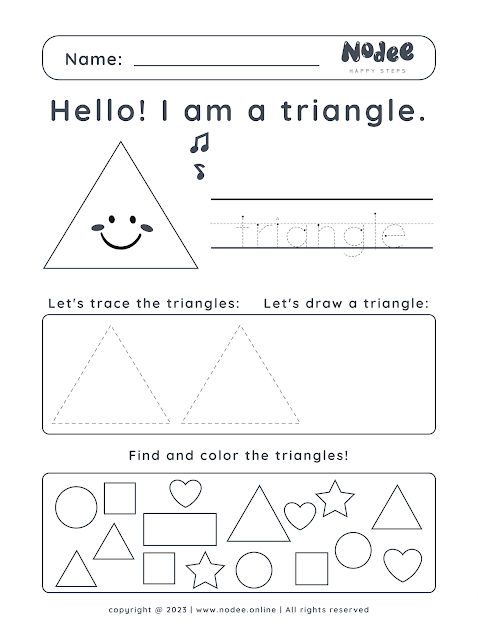# Triangle - Shapes Nursery Rhymes & worksheets - Learning Shapes for kids

Triangle shape song lyricsTriangle - Shapes Nursery Rhymes & worksheetsLearning Shapes for kids

Color Square shape worksheetTriangle - Shapes Nursery Rhymes & worksheetsLearning Shapes for kids

Black and white Square shape worksheetTriangle - Shapes Nursery Rhymes & worksheetsLearning Shapes for kids

Circle | Square

Do you want a great rhyme! We've effectively described the key characteristics of the triangle to be more clear for your kids.

A triangle

is a simple shape with three sides and three angles. It looks like a pizza slice or a musical instrument called a triangle!

Triangles come in different sizes and shapes, but they always have three sides and three angles. The three angles inside a triangle always add up to 180 degrees. This means that if you know two of the angles, you can figure out the third one by subtracting the sum of the two from 180.

There are different types of triangles based on the lengths of their sides and the angles between them. An equilateral triangle has three equal sides and three equal angles, each measuring 60 degrees. An isosceles triangle has two equal sides and two equal angles. A scalene triangle has three different sides and three different angles.

Triangles are found in many things around us.

For example, a slice of pizza is shaped like a triangle, and so is a traffic sign with a warning symbol. Triangles are also used in architecture to create strong and stable structures.

You can have fun with triangles by drawing them on paper and coloring them in different ways. You can also use triangles to make shapes like stars or houses. And if you want to challenge yourself, you can try to solve puzzles and games that involve triangles!

In conclusion, triangles are simple yet important shapes that are all around us. They have three sides and three angles and come in different sizes and shapes. So the next time you see a triangle, remember how special and versatile this shape is!